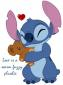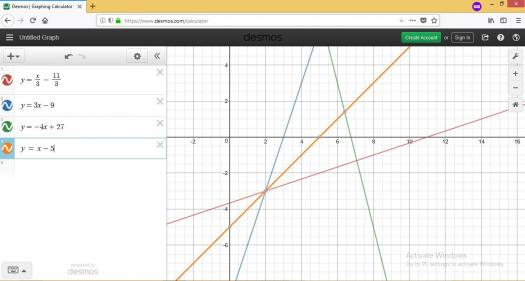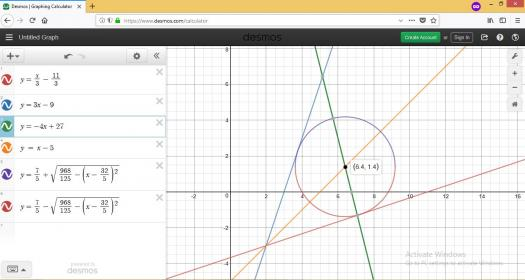# equation of a circle

2 posts / 0 new
Etteneaj Orachabequation of a circle

what is the equation of a circle touching the lines x-3y-11=0 and 3x-y-9=0 and having its center on the line 4x+y-27=0?

fitzmerl duronTo get the equation of the circle, minding those conditions, we need to make a figure...Now we need an acute angle bisector of equations $\color{red}{x-3y-11=0} \space or \space \color{red}{y = \frac{x}{3} - \frac{11}{3}}$ and $\color{blue}{3x-y-9=0} \space or \space \color{blue}{y = 3x-9}$ so we can locate the intersection of this acute angle bisector to the equation $4x+y-27=0 \space or \space y = -4x+27$. To get this acute angle bisector, do this:

Let $r_1$ be the distance from equation $\color{red}{x-3y-11=0} \space or \space \color{red}{y = \frac{x}{3} - \frac{11}{3}}$ to the point along the line $\color{green}{4x+y-27=0} \space or \color{green}{\space y = -4x+27}$ and let $r_2$ be the distance from equation $\color{blue}{3x-y-9=0} \space or \space \color{blue}{y = 3x-9}$ to the point along the line $\color{green}{4x+y-27=0} \space or \color{green}{\space y = -4x+27}$.

Recall that the distance from the line $Ax_1+By_1+C=0$ to a point $P(x,y)$ (or from a point $P(x,y)$ to a line $Ax+By+C=0$) is:

$$d = \frac{Ax_1+By_1+C}{\pm \sqrt{A^2 + B^2}}$$

and the sign of the radical $\sqrt{A^2 + B^2}$ must be opposite that of $C$.

Then....

$$r_1 = -r_2$$ $$\frac{3x-y-9}{+\sqrt{3^2+(-1)^2}} = -\frac{x-3y-11}{+\sqrt{1^2 + (-3)^2}}$$ $$3x-y-9 = -(x-3y-11)$$ $$3x-y-9 = -x+3y+11$$ $$4x-4y-20 =0$$ $$x-y-5=0$$

Getting the $y$, it becomes $y = x-5$. Intersection between $\color{orange}{x-y-5=0} \space or \space \color{orange}{y = x-5}$ and $\color{green}{4x+y-27=0} \space or \color{green}{\space y = -4x+27}$ is $C(x,y) = C(\frac{32}{5}, \frac{7}{5})$The distance from $C(x,y) = C(\frac{32}{5}, \frac{7}{5})$ to the line $\color{blue}{3x-y-9=0} \space or \space \color{blue}{y = 3x-9}$, which is the radius of this circle, is:

$$d = \frac{Ax_1+By_1+C}{\pm \sqrt{A^2 + B^2}}$$ $$d = \frac{3\left( \frac{32}{5}\right)+(-1)\left( \frac{7}{5}\right)-9}{\pm \sqrt{3^2 + (-1)^2}}$$ $$d = \frac{22\sqrt{10}}{25}$$

The distance from $C(x,y) = C(\frac{32}{5}, \frac{7}{5})$ to the line $\color{red}{x-3y-11=0} \space or \space \color{red}{y = \frac{x}{3} - \frac{11}{3}}$, which is the radius of this circle, is:

$$d = \frac{Ax_1+By_1+C}{\pm \sqrt{A^2 + B^2}}$$ $$d = \frac{1\left( \frac{32}{5}\right)+(-3)\left( \frac{7}{5}\right)-11}{\pm \sqrt{1^2 + (-3)^2}}$$ $$d = -\frac{22\sqrt{10}}{25}$$

Therefore, the radius of this circle is $\frac{22\sqrt{10}}{25}$ and the center of the circle is $C(x,y) = C(\frac{32}{5}, \frac{7}{5})$.

Ultimately, the equation of the circle is $\color{violet}{(x-\frac{32}{5})^2 + (y-\frac{7}{5})^2 = \left(\frac{22\sqrt{10}}{25}\right)^2}$.

Getting the $y$, it becomes $\color{violet}{y = \frac{7}{5} \pm \sqrt{\frac{968}{125} - \left(x - \frac{32}{5}\right)^2}}$Alternate solutions are encouraged.....

• Mathematics inside the configured delimiters is rendered by MathJax. The default math delimiters are $$...$$ and $...$ for displayed mathematics, and $...$ and $...$ for in-line mathematics.